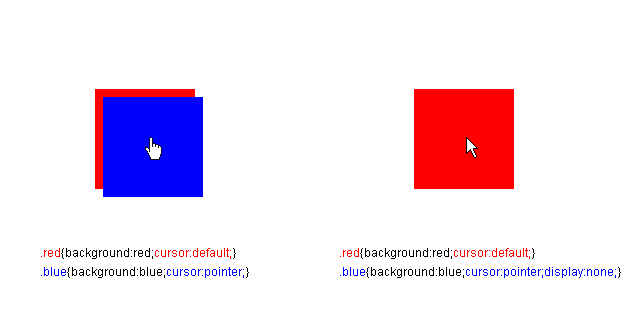# 那些CSS的细节问题(1)

## 1. 指针样式的变化## 2. 哪些CSS不占空间？

1. 绝对定位的元素：即 `position:absolute;` 以及 `position:fixed;`
2. 有偏移的相对定位的元素，指的是偏移后的元素不影响布局
3. `outlines`
4. Transforms变换属性偏移的元素
5. 各种阴影属性
6. 其他杂项：WebKit反射属性/IE私有滤镜/`text-decoration`/`display: none`

## 3. 实现彩虹的色彩

`box-shadow` 被用做边框或者渐变也不是什么新鲜事了，一年前我写的 CSS3 box-shadow 详解(2) 里曾经详细讨论过这些问题。由于多重阴影的出现，颜色何止是9种，那是要多少就有多少啊！于是，真正的第三个蛋疼的问题是：到底能有多少种颜色，到底，多重阴影的数量限制在哪里？

``````var r = g = b = 0;
for(var i = 0; i < 70000; i++){
console.log('0 ' + i + 'px 0 rgb('+r+','+g+','+b+'),');
if(r < 255){
r++;
}else{
r = 0;
if(g < 255){
g++;
}else{
g = 0;
if(b < 255){
b++
}else{
break;
}
}
}
}
``````

``````var r = 255;
var g = b = 0;
for(var i = 0; i < 256*5 - 4; i++){
console.log('0 ' + (i+1) + 'px 0 rgb('+r+','+g+','+b+'),');
if(g < 255 && r == 255 && b == 0){
g++;
}else if(g > 0 && r == 0 && b == 255){
g--;
}else if(r < 255 && g == 0 && b == 255){
r++;
}else if(r > 0 && g == 255 && b == 0){
r--;
}else if(b < 255 && r == 0 && g == 255){
b++;
}else if(b > 0 && r == 255 && g == 0){
b--;
}
}
``````

Disqus提供评论支持，如果评论长时间未加载，请飞跃长城。